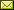## Weighted Linear Dynamic Logic

 Manfred Droste (Leipzig, Germany) George Rahonis (Thessaloniki, Greece)

 We introduce a weighted linear dynamic logic (weighted LDL for short) and show the expressive equivalence of its formulas to weighted rational expressions. This adds a new characterization for recognizable series to the fundamental Schützenberger theorem. Surprisingly, the equivalence does not require any restriction to our weighted LDL. Our results hold over arbitrary (resp. totally complete) semirings for finite (resp. infinite) words. As a consequence, the equivalence problem for weighted LDL formulas over fields is decidable in doubly exponential time. In contrast to classical logics, we show that our weighted LDL is expressively incomparable to weighted LTL for finite words. We determine a fragment of the weighted LTL such that series over finite and infinite words definable by LTL formulas in this fragment are definable also by weighted LDL formulas.

In Domenico Cantone and Giorgio Delzanno: Proceedings of the Seventh International Symposium on Games, Automata, Logics and Formal Verification (GandALF 2016), Catania, Italy, 14-16 September 2016, Electronic Proceedings in Theoretical Computer Science 226, pp. 149–163.
Published: 13th September 2016.

 ArXived at: http://dx.doi.org/10.4204/EPTCS.226.11 bibtex PDF
References in reconstructed bibtex, XML and HTML format (approximated).
 Comments and questions to:eptcs@eptcs.org For website issues:webmaster@eptcs.org